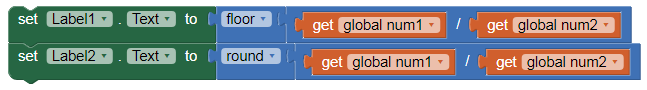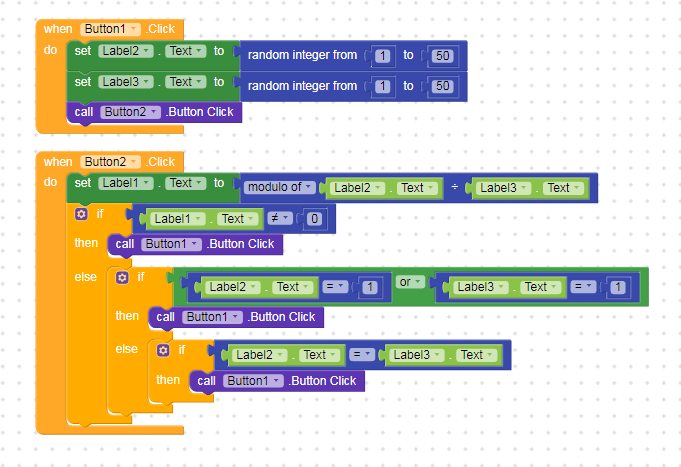# I want two numbers in which the first number can be divided into the second number

i want to get value in (1,2,3,4…98,99,100)

but i use block as image got value is ( 0.002, 1.132, 5.88, 2.33)

So my question is how to i set block to got value = (1,2,3,4…98,99,100).

global number 1 = random from 100 to 200
global number 2 = random from 1 to 100

button2.click
Math.Ceiling ( number 1 / number 2)

global number 1 = random from 100 to 200 = 119
global number 2 = random from 1 to 100 = 29

Than result is = 4.1034482758

but i want every time result without (.) means divisible

Between 1 and 100 there are several prime numbers that are not divisible. What’s up with them? So you need to round up / down …

what is round up / down … ?

and i don’t want random 1 to 100 I want two numbers in which the first number can be divided into the second numbermeans label 1 is divisible label 2 ?

Okay, so you’ll have to use “modulo” block. If result is 0, then its divisible, else isn’t and you have to find another pair

thanks its working i use block as below image1 Like

Glad its working nowif i use this method to app hang and say runtime error stack 8 mb

I think that means you have a logic error that’s causing memory issues. The “modulo” should not cause this error

but after i apply this block in my application after than showing error other than working fine

You created an endless loop… When should your logic end? Currently it always switches between the 2 buttons…
Taifun

2 Likes

so what is the solution ?

Break loopusing break block after getting right answer

after getting right answer i break see in block

Where is break block

You will find this block under control section

1 Like